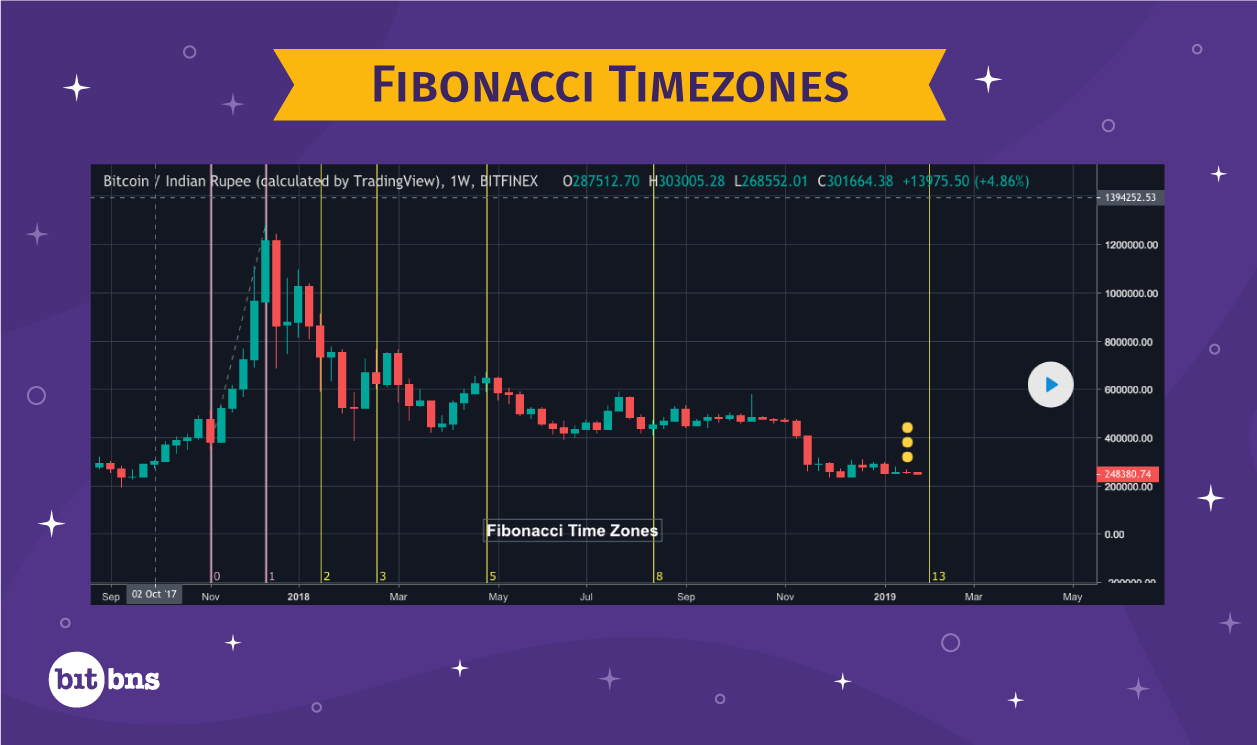# Fibonacci Tools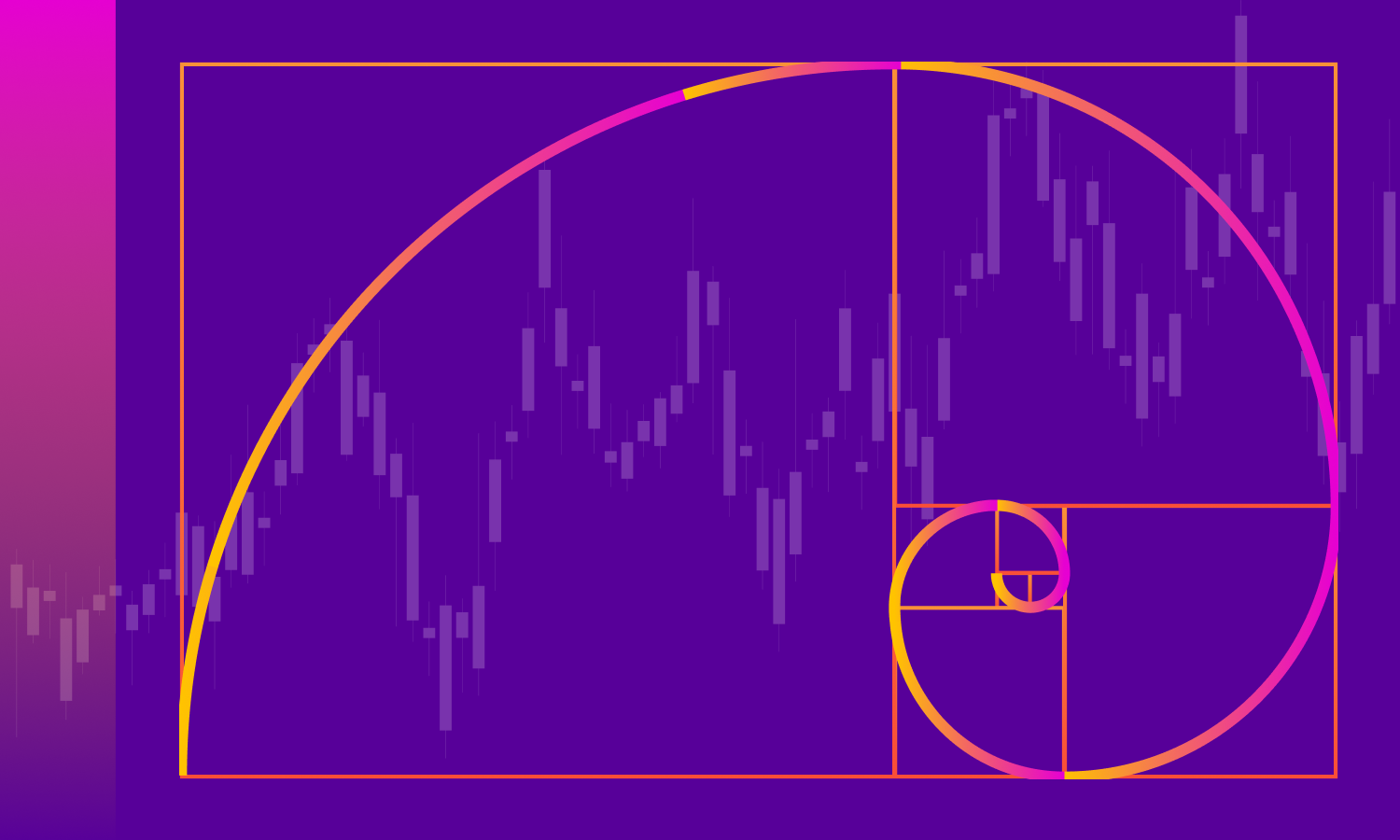### Fibonacci

Fibonacci Tools are TA tools that are based on the Fibonacci Series of numbers. Fibonacci Series is an interesting series of numbers, present in fascinating examples of nature and everything around us.

Fibonacci Series starts from 1 and is derived by adding any number in the series to the preceding number to find the next number in the series.

0, 1, 1, 2, 3, 5, 8, 13, 21, 34, 55, 89……..

Notice that
1+0 = 1
1+1 = 2
2+1 = 3
3+2 = 5
5+3 = 8
8+5 = 13
13+8 = 21
21+13 = 34

And it goes on.
There are many interesting properties of Fibonacci Series, but the ones we will focus on here are Fibonacci ratios, which are derived by dividing a number in the series by the numbers that follow. Different ratios can be extracted when selecting the denominator (the number by which we divide the other number) by skipping one number in the series each time we select a new denominator.

For example –

Fibonacci Series – 0, 1, 1, 2, 3, 5, 8, 13, 21, 34, 55, 89……..

When the immediate next number is selected as denominator
55/89 = 0.6179
34/55 = 0.6181
21/34 = 0.6176
13/21 = 0.6190

When one number is skipped to select the denominator

34/89 = 0.3820
21/55 = 0.3818
13/34 = 0.3823
08/21 = 0.3809

When two numbers are skipped to select the denominator

21/89 = 0.2359
13/55 = 0.2363
08/34 = 0.2352

In the same fashion, we keep increasing the gap by one to get a new ratio.
The ratios derived can be expressed in percentages as well, which gives us these values:

100%, 61.8%, 38.2%, 23.6%, 14.6%, 9%, 5.6%, and 0%.

### Fibonacci Retracement

Often, it has been observed that the prices retract after rallying up or diving down, following a pattern derived from Fibonacci ratios. Every time the price of an asset moves up or down, it corrects itself or retraces by some degree. These degrees of retracement have been found to match Fibonacci ratios. Thus, a trader can predict the degree of retracement or at least the support or resistance level where the advance or decline may stall.
Here is a chart showing Fibonacci Retracement: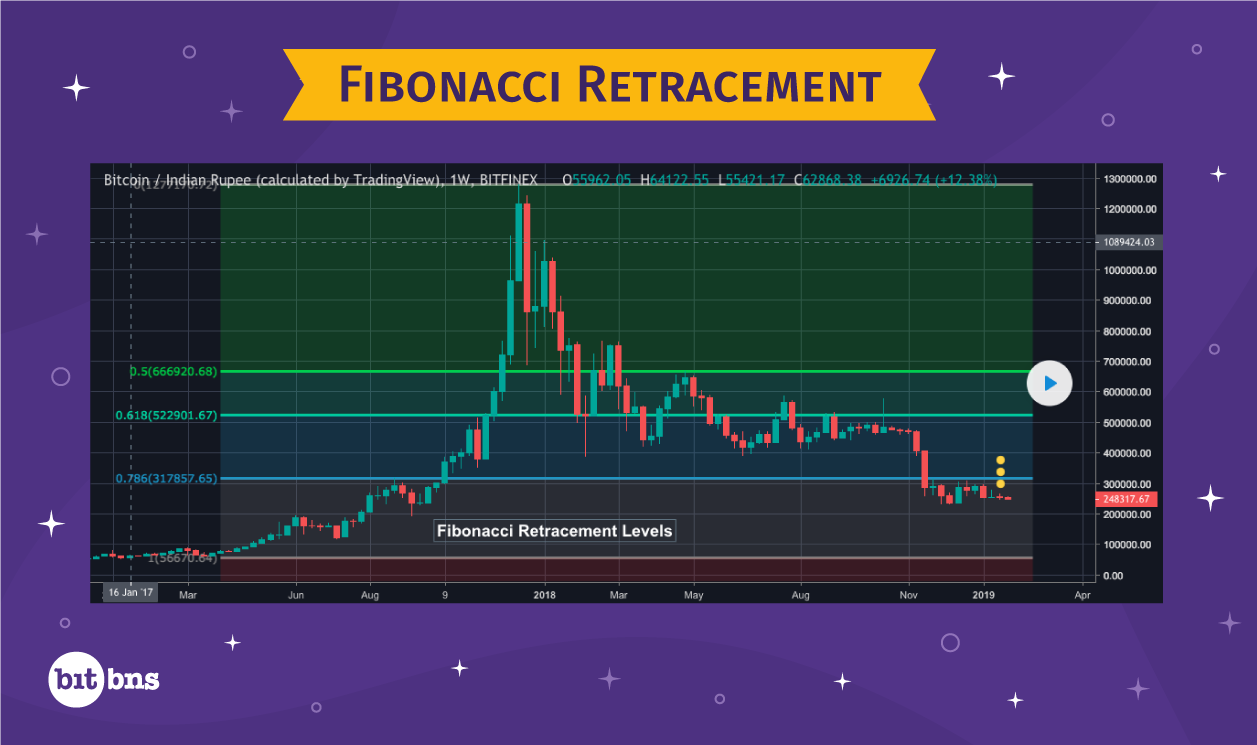### Fibonacci Fan

A Fibonacci Fan is a technical analysis tool which, when applied to a chart, creates support and resistance lines based on the Fibonacci ratios or percentages. The most unique thing about Fibonacci Fans is that they can be applied to a chart of any time period, in a very intuitive way. Usually, various technical analysis tools are said to be effective in charts with specific time periods due to the different degrees of fluctuations in different time periods; shorter time periods being more turbulent and longer time periods being more stable.
But unlike other TA tools, Fibonacci Fans apply to charts of any time period.
A Fibonacci Fan is created either from the highest peak or the lowest trough. Most of the traders would use trading software to apply Fibonacci Fans in order to find support and resistance levels at a particular point in time. Each resistance or support level is marked at one of the Fibonacci Ratios that we discussed earlier.
Take this chart for instance: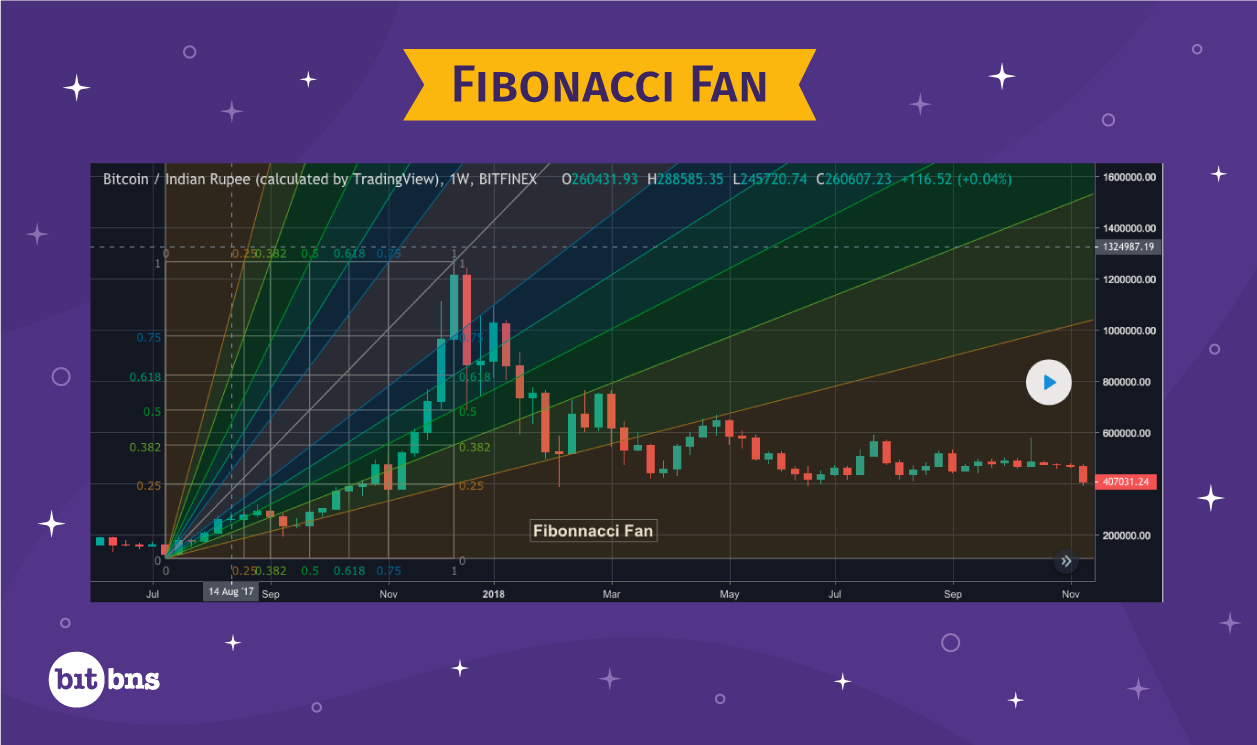### Fibonacci Arcs

Another way to draw support and resistance levels is Fibonacci Arcs. It is also a technical analysis tool, which draws arcs between the highest peak and the lowest trough on a connecting line, called the Base Line. The arcs dissect the Base Line at points that are determined by Fibonacci ratios. The first arc is drawn at 38.2% of the base line, the second is drawn at 50% and the third is drawn at 61.8%. Like Fibonacci Fans, Fibonacci Arcs also create support and resistance levels, which traders can use to make trading decisions.

The length of the base line depends on the price movement and timeframe of reference. If the price movement is huge and the time period is long, the width of the arcs would be more due to a longer base line. If the price movement and time period are less, the arcs would be more packed and narrow due to a shorter base line.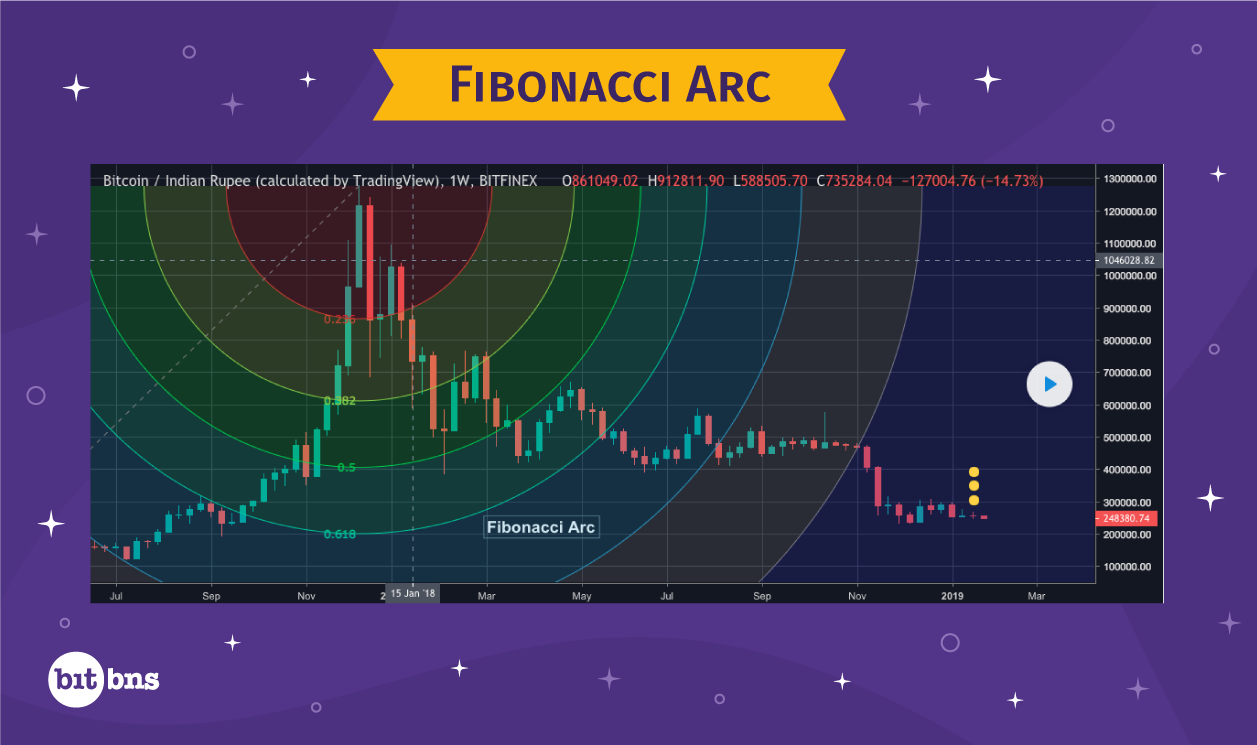### Fibonacci Time Zones or Extensions

Fibonacci Time Zone, also called Fibonacci Extension, is a charting tool under technical analysis, which is used to create different time zones that mark the rally or fall in price movements. Fibonacci Time Zones imply that time zones created out of Fibonacci numbers have a significance in price movements and that prices move from time zone to time zone.

Fibonacci Time Zones are easy to figure out and anyone can do it. Wondering how? Keep reading.

Let us take a look again at the Fibonacci series:

0,1,1,2,3,5,8,13,21,34,55,89,144,233, 377 and so on.

To find the next number in the Fibonacci series, simply add the last number to its previous number. For example – 233+144 = 377.

Fibonacci Time Zones start from a major peak or trough and are marked at distances that grow relatively, with the growing differences between Fibonacci numbers.
Based on the above information, here are the first 10 Fibonacci Time Zones:

1st Time Zone = 0 to 1
2nd Time Zone = 1 to 1
3rd Time Zone = 1 to 2
4th Time Zone = 2 to 3
5th Time Zone = 3 to 5
6th Time Zone = 5 to 8
7th Time Zone = 8 to 13
8th Time Zone = 13 to 21
9th Time Zone = 21 to 34
10th Time Zone = 34 to 55

Beyond this, I assume you can calculate the next time zone by adding the 34 and 55, and so on.

Now let’s take a look at this chart that applies Fibonacci Time Zones or Extensions to figure out price movements: